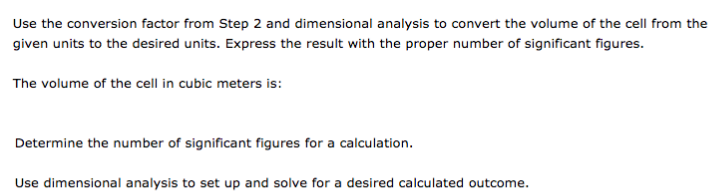# Problem: Use the conversion factor from Step 2 and dimensional analysis to convert the volume of the cell from the given units to the desired units. Express the result with the proper number of significant figures. The volume of the cell in cubic meters is: Determine the number of significant figures for a calculation. Use dimensional analysis to set up and solve for a desired calculated outcome.

###### FREE Expert Solution
86% (249 ratings)###### Problem Details

Use the conversion factor from Step 2 and dimensional analysis to convert the volume of the cell from the given units to the desired units. Express the result with the proper number of significant figures.

The volume of the cell in cubic meters is:

Determine the number of significant figures for a calculation.

Use dimensional analysis to set up and solve for a desired calculated outcome.